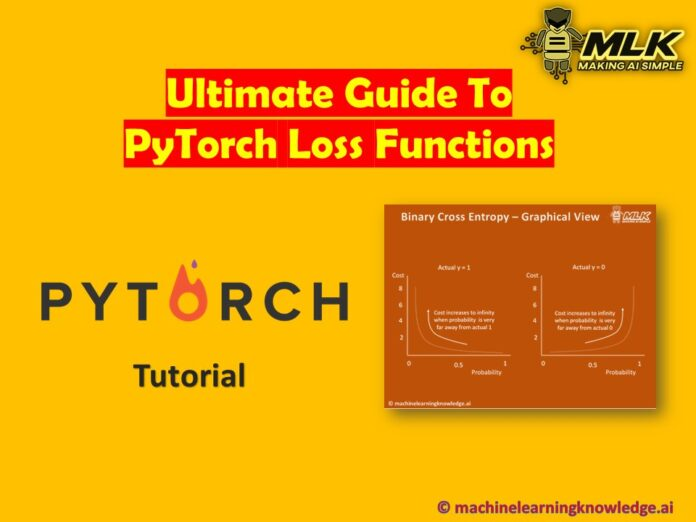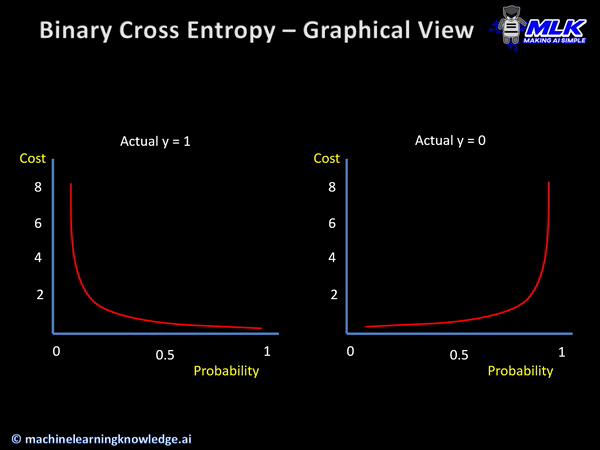# Ultimate Guide to PyTorch Loss Functions### 2. PyTorch Loss Functions for Classification

Let us now understand the PyTorch loss functions for classification problem that is generally calculated using probabilistic losses.##### When could it be used?

This function can be used for binary classification.

##### Syntax

Below is the syntax of the Cross-Entropy loss function in PyTorch.

##### Example of Hinge Embedding Loss in PyTorch
The below example shows how we can implement Hinge Embedding Loss in PyTorch.